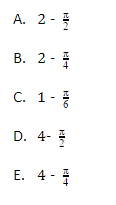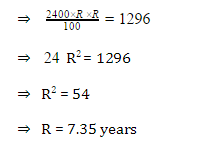# GMAT Quant: Word ProblemsDiscount

Question: On Rs. 2500 due 5 months the true discount is Rs. 250. Calculate the rate percent:

1. 26.67%
2. 30.33%
3. 33%
4. 15%

Solution: P. W. = Rs. (2500 – 250) = Rs. 2250

Therefore, SI on Rs. 2250 for 5 month period is Rs. 250.

$\large Rate = \left [ \frac{100 \times 250}{2250 \times \frac{5}{12}} \right ] \% = 26.67 \%$

Geometry Problems

Question: A circle is inscribed in a square with the diagonal of 2 centimeters. What is the area of the square that is not occupied by the circle?Solution: Area of square $= \frac{(Diagonal)^2}{2}$

= $= \frac{(2)^2}{2}$ $= 2$

Next, since the diagonal of the square equals to 2 centimeters, then the side of the square equals to-

a2 + a2 = 22

2 a 2 = 4

a2 = 2

$a = \sqrt{2}$

The radius of inscribed circle will be half of the side of the square, so it will be $\frac{\sqrt{2}}{2}$ $Area, \; A = \pi r^{2}$ $\pi \times \frac{2}{4}$ $\frac{\pi}{2}$

The radius of inscribed circle will be half of the side of the square, so it will be.

Area,

Area not occupied by circle is $2cm^{2}$.

Interest Problems

Simple Interest

Question: Rita took a loan of Rs. 2400 on simple interest. The loan is taken for as many years as the interest rate is. If the amount she paid is Rs. 1296 by the end of the loan period, then what is the rate of interest at which she took the loan?

1. 3.6
2. 7.35
3. 18
4. Cannot be determined
5. None of these

Solution: Let rate = R% and time = R years.

Then according to the formula of simple interest,Compound Interest

Question: The compound interest on Rs. 50,000 at 8% per annum is Rs. 8320. Calculate the time period in years:

1. 5
2. 3
3. 2
4. 1

Solution: Amount = (50,000 + 8320) = 58, 320

Let the time be T years.

So, using the formula of compound Interest.• So, n = 2 years.

Measurement Problems

Question: Yard is to inch then the quart is to

1. gallon
2. ounce
3. milk
4. liquid

Solution: The answer is B, as yard is a measurement unit larger than the inch; similarly quart is a larger measurement unit then the ounce, whereas, gallon is a larger quantity than quart. However, milk and liquid is not a measuring unit.

Question: Embarrassed is to humiliated as frightened is to

1. terrified
2. agitated
3. courageous
4. reckless

People will feel embarrassed, if they get humiliated. Those people, who are frightened, will be terrified. Agitated people are not frightened people. Courageous and reckless are not the state of fear.

Mixture Problems

Question: A cylinder is filled with a mixture of two miscible liquids X and Y is a ratio of 7: 5. If 9 liters of mixture is drawn and filled with liquid Y, then the ratio of X:Y becomes 7: 9. How many liters of liquid X was present in the cylinder initially?

1. 10
2. 20
3. 21
4. 25

Solution: Suppose, initially the cylinder contained 7x and 5x of mixture X and Y respectively.Profit

Question: Ram sells one radio at a profit of 20% for a cost of Rs. 840 and another radio at a loss of 4% at the price of Rs. 960. Calculate his total percentage of profit or lossSets and Venn Diagrams

Question: A school has 63 students studying Physics, Chemistry and Biology. 33 study Physics, 25 studies Chemistry and 26 Biology. 10 study both Physics and Chemistry, 9 study Biology and Chemistry, while 8 study both Physics and Biology. Equal numbers study all three subjects as those who learn none of the three. How many study all the three subjects?

1. 2
2. 3
3. 5
4. 7
5. 8

Solution: Let us first draw the Venn diagram for this question:From the diagram, 3 students study all the three subjects, Therefore the correct option is (B).

Time Speed (Rate) Problems

Question:

Jack and Jill set out together on bicycle travelling at 15 and 12 miles per hour, respectively. After 40 minutes, Jack stops to fix a flat tire. If it takes Jack an hour to fix the flat tire and Jill continues to ride during this time, how many hours will it take Jack to catch up to Jill assuming he resumes his ride at 15 miles per hour?

(Consider Jack’s deceleration/ acceleration before/ after the flat tire to be negligible)

1. 3
2. 3.33
3. 3.5
4. 4
5. 4.5

Solution:

Distance travelled by Jack in 40 mins $= \frac{40}{60} \times 15 = 10 \; miles$

Distance travelled by Jill in 40 mins $= \frac{40}{60} \times 12 = 8 \; miles$

When Jack stops to fix the flat tire, Jill is still travelling.

Distance covered by Jill in 1 Hour = 12 miles

Total distance covered by Jill in 1 hour 40 minutes = 20 miles

Distance between Jack and Jill after 1 hour and 40 mins = (20 – 10) miles = 10 miles

Since, Jack and Jill are travelling in the same direction, their relative velocity = 15 – 12 = 3 miles/hour.

Time taken by Jack to catch up with Jill $= \frac{10}{4} = 3.33 \; hours$

Hence, the correct answer is B.

Work Problems

Question: Tom working alone can paint a room in 6 hours. Peter and John, working independently, can paint the same room in 3 hours and 2 hours respectively. Tom starts painting the room and works on his own for one hour. He is then joined by Peter and they work together for an hour. Finally, John joins them and the three of them work together to finish the room, each one working at his respective rate. What fraction of the whole job was done by Peter?

1. 1/9
2. 1/6
3. 1/3
4. 7/18
5. 4/9

Solution: Let’s work with smart numbers here!

Let the total work be 24 units. Then using Rate we have

Tom’s rate of work = 4 units per hour [Tom paints the room in 6 hour, working alone]

Peter’s rate of work = 8 units per hour [Peter paints the room in 3 hour, working alone]

John’s rate of work = 12 units per hour [John paints the room in 2 hour, working alone]

First hour, Tom working alone, amount of work done = 4 units

Second hour, Tom and Peter working together, amount of work done = 4+ 8 = 12

Total work done in 2 hours = 16

Amount of work left = 24 – 16 = 8

Tom, Peter and John work together to complete the remaining work.

Their combined rate of work = 4 + 8 + 12 = 24 units per hour.

Time taken to complete the remaining work = 8/24 = 1/3 hour

Therefore, amount of work done by Peter in third hour = 8/3

Total amount of work done by Peter = 8 + 8/3 = 32/3

Fraction of total work done by Peter = (32/3)/24 = 4/9

Hence, the correct answer is (C).

We’ll be glad to help you in your GMAT preparation journey. You can ask for any assistance related to GMAT and MBA from us by calling us at +918884544444. You can write to us at gmat@byjus.com.